Скачать презентацию UNIVERSITY OF MASSACHUSETTS Dept of Electrical Computer

147d0aa779fe797ec9bfaf79510d41b2.ppt

• Количество слайдов: 20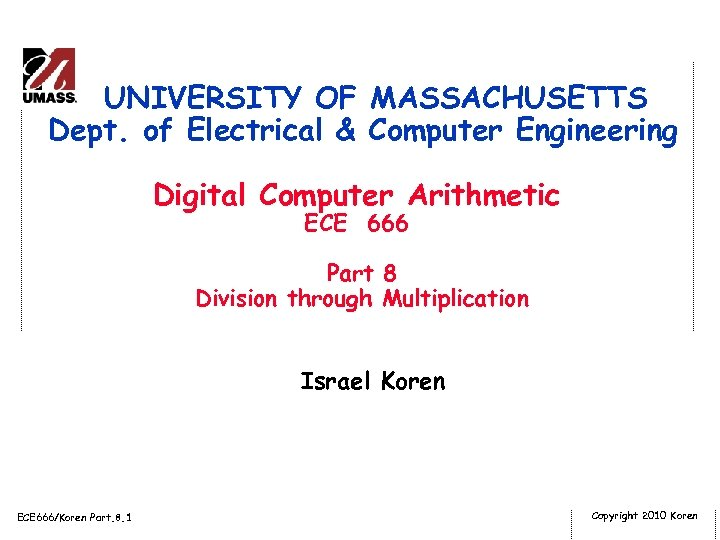UNIVERSITY OF MASSACHUSETTS Dept. of Electrical & Computer Engineering Digital Computer Arithmetic ECE 666 Part 8 Division through Multiplication Israel Koren ECE 666/Koren Part. 8. 1 Copyright 2010 Koren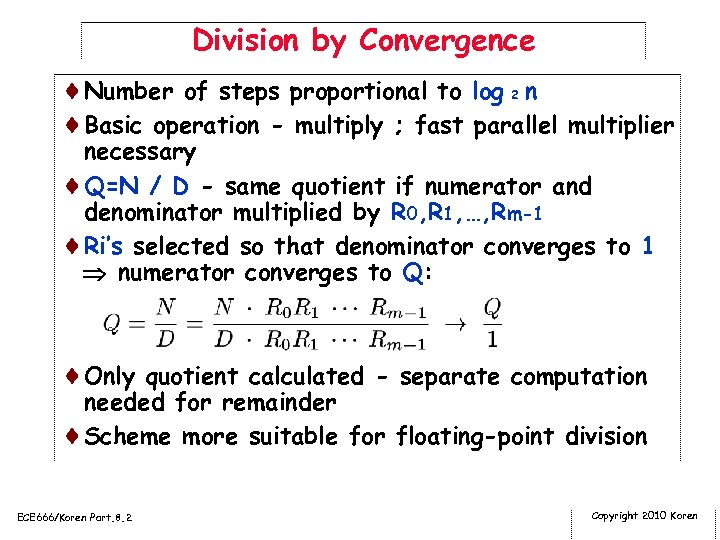Division by Convergence ¨Number of steps proportional to log 2 n ¨Basic operation - multiply ; fast parallel multiplier necessary ¨Q=N / D - same quotient if numerator and denominator multiplied by R 0, R 1, …, Rm-1 ¨Ri’s selected so that denominator converges to 1 numerator converges to Q: ¨Only quotient calculated - separate computation needed for remainder ¨Scheme more suitable for floating-point division ECE 666/Koren Part. 8. 2 Copyright 2010 Koren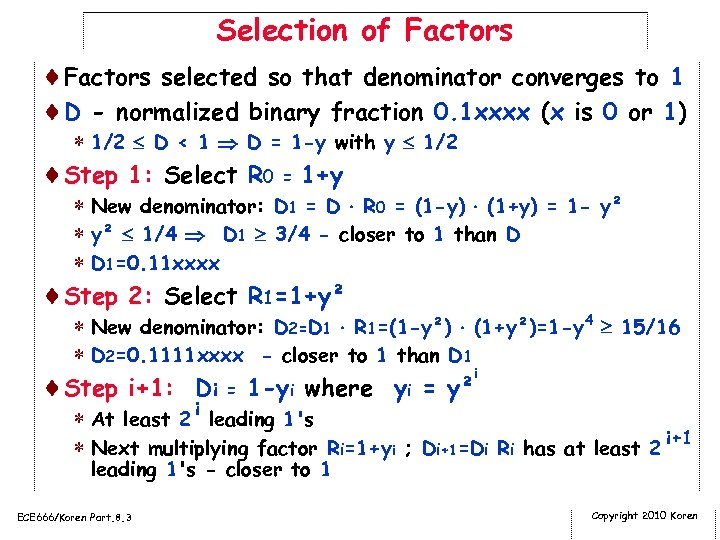Selection of Factors ¨Factors selected so that denominator converges to 1 ¨D - normalized binary fraction 0. 1 xxxx (x is 0 or 1) * 1/2 D < 1 D = 1 -y with y 1/2 ¨Step 1: Select R 0 = 1+y * New denominator: D 1 = D R 0 = (1 -y) (1+y) = 1 - y² * y² 1/4 D 1 3/4 - closer to 1 than D * D 1=0. 11 xxxx ¨Step 2: Select R 1=1+y² * New denominator: D 2=D 1 R 1=(1 -y²) (1+y²)=1 -y 4 15/16 * D 2=0. 1111 xxxx - closer to 1 than D 1 i ¨Step i+1: Di = 1 -yi where yi = y² * At least 2 i leading 1's * Next multiplying factor Ri=1+yi ; Di+1=Di Ri has at least 2 i+1 leading 1's - closer to 1 ECE 666/Koren Part. 8. 3 Copyright 2010 Koren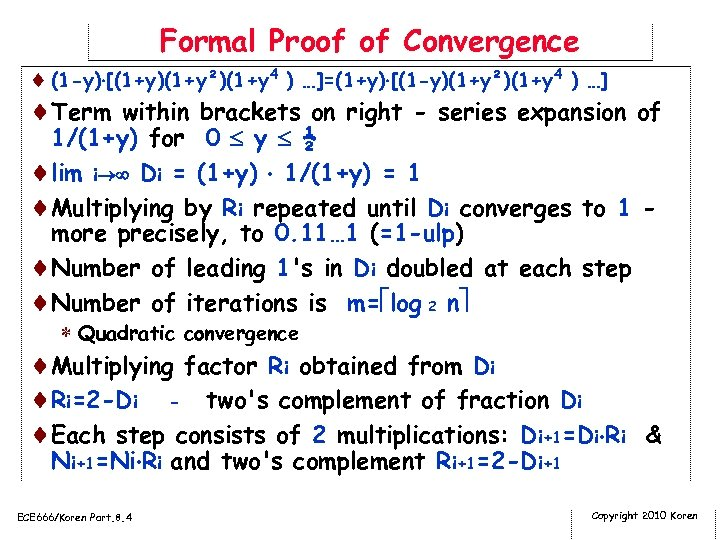Formal Proof of Convergence ¨ (1 -y) [(1+y)(1+y²)(1+y 4 ) …]=(1+y) [(1 -y)(1+y²)(1+y 4 ) …] ¨Term within brackets on right - series expansion of 1/(1+y) for 0 y ½ ¨lim i Di = (1+y) 1/(1+y) = 1 ¨Multiplying by Ri repeated until Di converges to 1 more precisely, to 0. 11… 1 (=1 -ulp) ¨Number of leading 1's in Di doubled at each step ¨Number of iterations is m= log 2 n * Quadratic convergence ¨Multiplying factor Ri obtained from Di ¨Ri=2 -Di - two's complement of fraction Di ¨Each step consists of 2 multiplications: Di+1=Di Ri & Ni+1=Ni Ri and two's complement Ri+1=2 -Di+1 ECE 666/Koren Part. 8. 4 Copyright 2010 Koren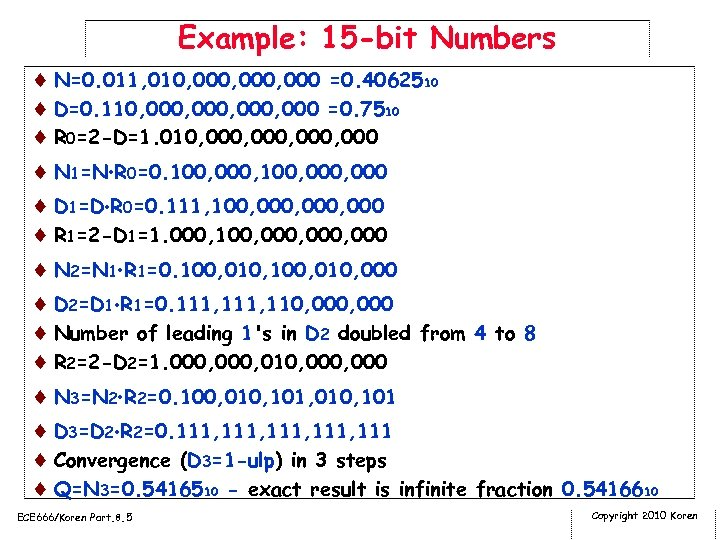Example: 15 -bit Numbers ¨ N=0. 011, 010, 000, 000 =0. 4062510 ¨ D=0. 110, 000, 000 =0. 7510 ¨ R 0=2 -D=1. 010, 000, 000 ¨ N 1=N R 0=0. 100, 000, 000 ¨ D 1=D R 0=0. 111, 100, 000, 000 ¨ R 1=2 -D 1=1. 000, 100, 000, 000 ¨ N 2=N 1 R 1=0. 100, 010, 000 ¨ D 2=D 1 R 1=0. 111, 110, 000 ¨ Number of leading 1's in D 2 doubled from 4 to 8 ¨ R 2=2 -D 2=1. 000, 010, 000 ¨ N 3=N 2 R 2=0. 100, 010, 101 ¨ D 3=D 2 R 2=0. 111, 111 ¨ Convergence (D 3=1 -ulp) in 3 steps ¨ Q=N 3=0. 5416510 - exact result is infinite fraction 0. 5416610 ECE 666/Koren Part. 8. 5 Copyright 2010 Koren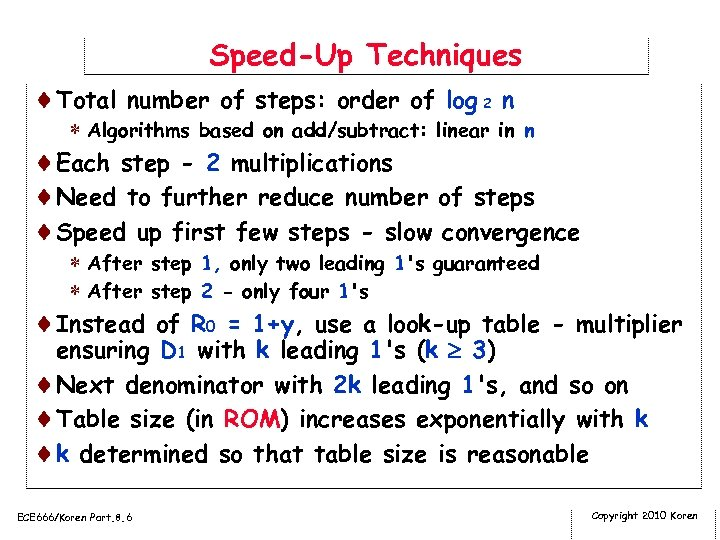Speed-Up Techniques ¨Total number of steps: order of log 2 n * Algorithms based on add/subtract: linear in n ¨Each step - 2 multiplications ¨Need to further reduce number of steps ¨Speed up first few steps - slow convergence * After step 1, only two leading 1's guaranteed * After step 2 - only four 1's ¨Instead of R 0 = 1+y, use a look-up table - multiplier ensuring D 1 with k leading 1's (k 3) ¨Next denominator with 2 k leading 1's, and so on ¨Table size (in ROM) increases exponentially with k ¨k determined so that table size is reasonable ECE 666/Koren Part. 8. 6 Copyright 2010 Koren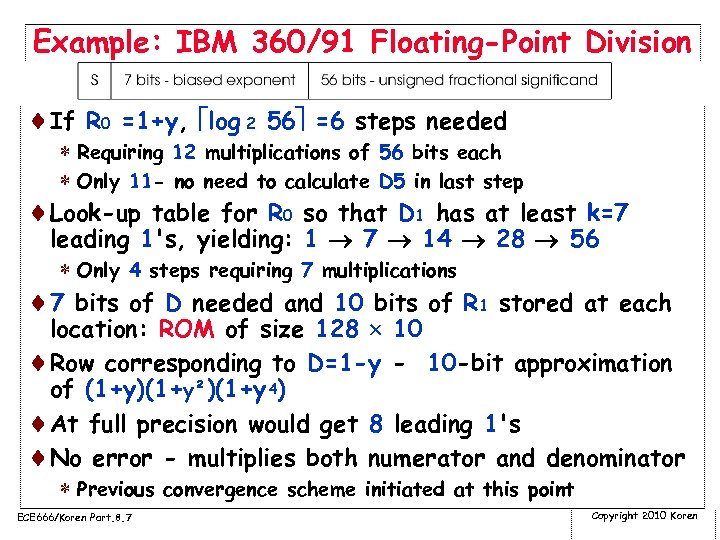Example: IBM 360/91 Floating-Point Division ¨If R 0 =1+y, log 2 56 =6 steps needed * Requiring 12 multiplications of 56 bits each * Only 11 - no need to calculate D 5 in last step ¨Look-up table for R 0 so that D 1 has at least k=7 leading 1's, yielding: 1 7 14 28 56 * Only 4 steps requiring 7 multiplications ¨ 7 bits of D needed and 10 bits of R 1 stored at each location: ROM of size 128 10 ¨Row corresponding to D=1 -y - 10 -bit approximation of (1+y)(1+y²)(1+y 4) ¨At full precision would get 8 leading 1's ¨No error - multiplies both numerator and denominator * Previous convergence scheme initiated at this point ECE 666/Koren Part. 8. 7 Copyright 2010 Koren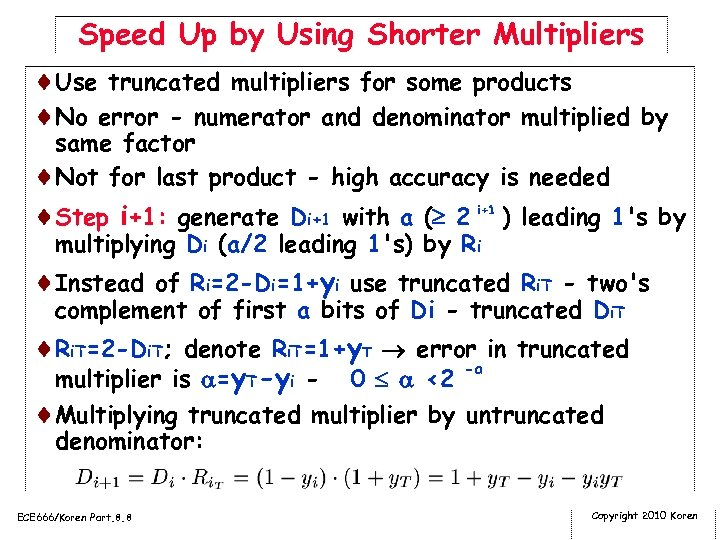Speed Up by Using Shorter Multipliers ¨Use truncated multipliers for some products ¨No error - numerator and denominator multiplied by same factor ¨Not for last product - high accuracy is needed ¨Step i+1: generate Di+1 with a ( 2 i+1 ) leading 1's by multiplying Di (a/2 leading 1's) by Ri ¨Instead of Ri=2 -Di=1+yi use truncated Ri. T - two's complement of first a bits of Di - truncated Di. T ¨Ri. T=2 -Di. T; denote Ri. T=1+y. T error in truncated -a multiplier is =y. T-yi - 0 <2 ¨Multiplying truncated multiplier by untruncated denominator: ECE 666/Koren Part. 8. 8 Copyright 2010 Koren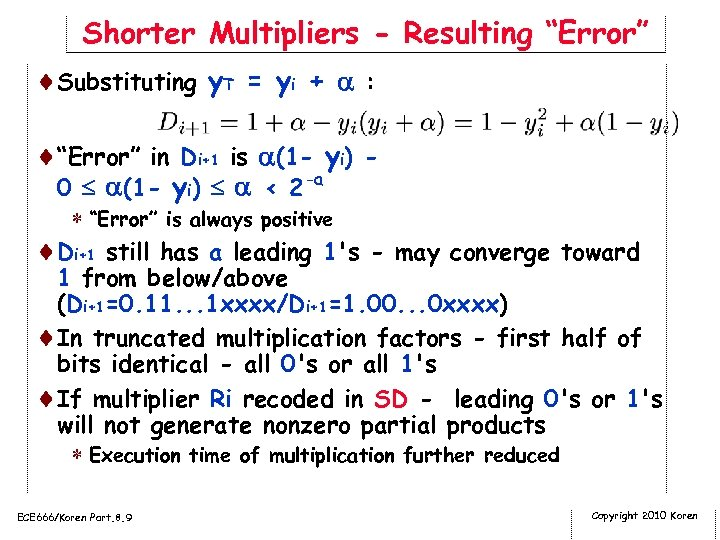Shorter Multipliers - Resulting “Error” ¨Substituting y. T = yi + : ¨“Error” in Di+1 is (1 - yi) 0 (1 - yi) < 2 -a * “Error” is always positive ¨Di+1 still has a leading 1's - may converge toward 1 from below/above (Di+1=0. 11. . . 1 xxxx/Di+1=1. 00. . . 0 xxxx) ¨In truncated multiplication factors - first half of bits identical - all 0's or all 1's ¨If multiplier Ri recoded in SD - leading 0's or 1's will not generate nonzero partial products * Execution time of multiplication further reduced ECE 666/Koren Part. 8. 9 Copyright 2010 Koren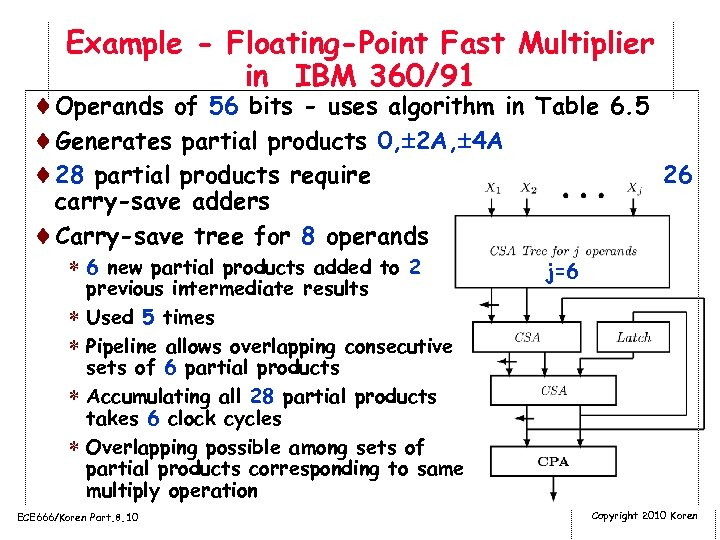Example - Floating-Point Fast Multiplier in IBM 360/91 ¨Operands of 56 bits - uses algorithm in Table 6. 5 ¨Generates partial products 0, 2 A, 4 A ¨ 28 partial products require 26 carry-save adders ¨Carry-save tree for 8 operands * 6 new partial products added to 2 previous intermediate results * Used 5 times * Pipeline allows overlapping consecutive sets of 6 partial products * Accumulating all 28 partial products takes 6 clock cycles * Overlapping possible among sets of partial products corresponding to same multiply operation ECE 666/Koren Part. 8. 10 j=6 Copyright 2010 Koren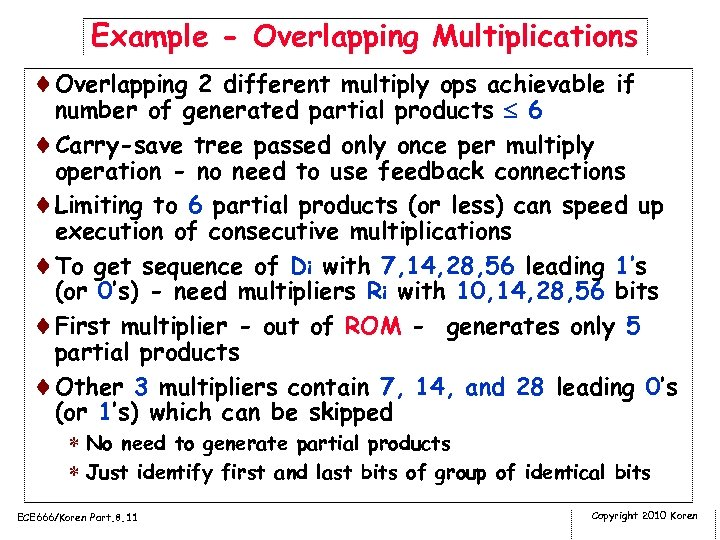Example - Overlapping Multiplications ¨Overlapping 2 different multiply ops achievable if number of generated partial products 6 ¨Carry-save tree passed only once per multiply operation - no need to use feedback connections ¨Limiting to 6 partial products (or less) can speed up execution of consecutive multiplications ¨To get sequence of Di with 7, 14, 28, 56 leading 1’s (or 0’s) - need multipliers Ri with 10, 14, 28, 56 bits ¨First multiplier - out of ROM - generates only 5 partial products ¨Other 3 multipliers contain 7, 14, and 28 leading 0’s (or 1’s) which can be skipped * No need to generate partial products * Just identify first and last bits of group of identical bits ECE 666/Koren Part. 8. 11 Copyright 2010 Koren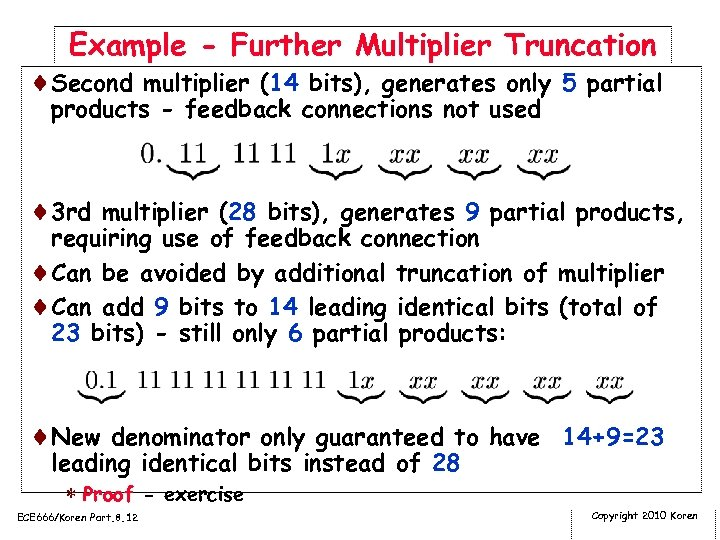Example - Further Multiplier Truncation ¨Second multiplier (14 bits), generates only 5 partial products - feedback connections not used ¨ 3 rd multiplier (28 bits), generates 9 partial products, requiring use of feedback connection ¨Can be avoided by additional truncation of multiplier ¨Can add 9 bits to 14 leading identical bits (total of 23 bits) - still only 6 partial products: ¨New denominator only guaranteed to have 14+9=23 leading identical bits instead of 28 * Proof - exercise ECE 666/Koren Part. 8. 12 Copyright 2010 Koren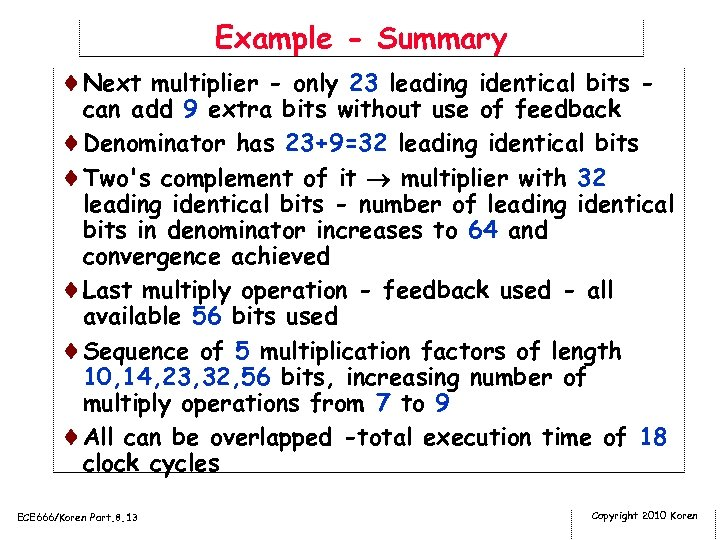Example - Summary ¨Next multiplier - only 23 leading identical bits - can add 9 extra bits without use of feedback ¨Denominator has 23+9=32 leading identical bits ¨Two's complement of it multiplier with 32 leading identical bits - number of leading identical bits in denominator increases to 64 and convergence achieved ¨Last multiply operation - feedback used - all available 56 bits used ¨Sequence of 5 multiplication factors of length 10, 14, 23, 32, 56 bits, increasing number of multiply operations from 7 to 9 ¨All can be overlapped -total execution time of 18 clock cycles ECE 666/Koren Part. 8. 13 Copyright 2010 Koren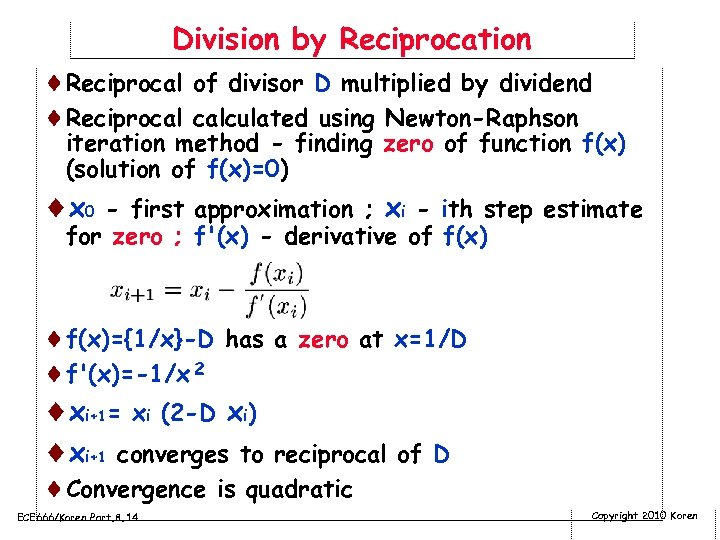Division by Reciprocation ¨Reciprocal of divisor D multiplied by dividend ¨Reciprocal calculated using Newton-Raphson iteration method - finding zero of function f(x) (solution of f(x)=0) ¨ x 0 - first approximation ; xi - ith step estimate for zero ; f'(x) - derivative of f(x) ¨f(x)={1/x}-D has a zero at x=1/D ¨f'(x)=-1/x 2 ¨xi+1= xi (2 -D xi) ¨xi+1 converges to reciprocal of D ¨Convergence is quadratic ECE 666/Koren Part. 8. 14 Copyright 2010 Koren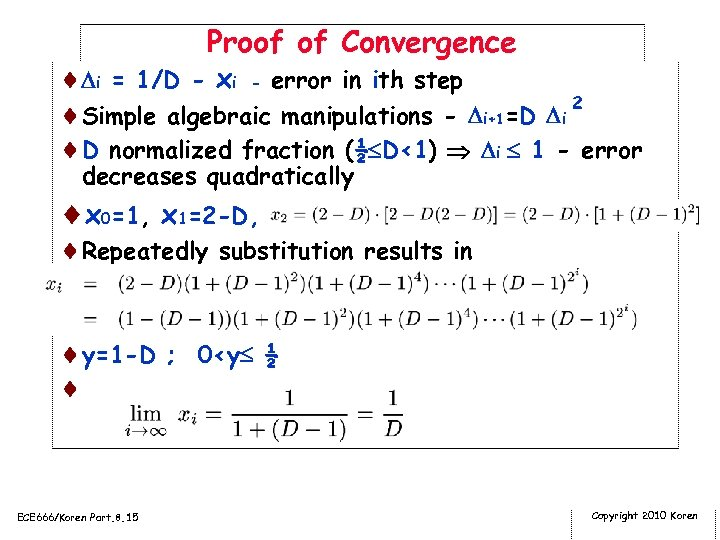Proof of Convergence ¨ i = 1/D - xi - error in ith step ¨Simple algebraic manipulations - i+1=D i 2 ¨D normalized fraction (½ D<1) i 1 - error decreases quadratically ¨x 0=1, x 1=2 -D, ¨Repeatedly substitution results in ¨y=1 -D ; 0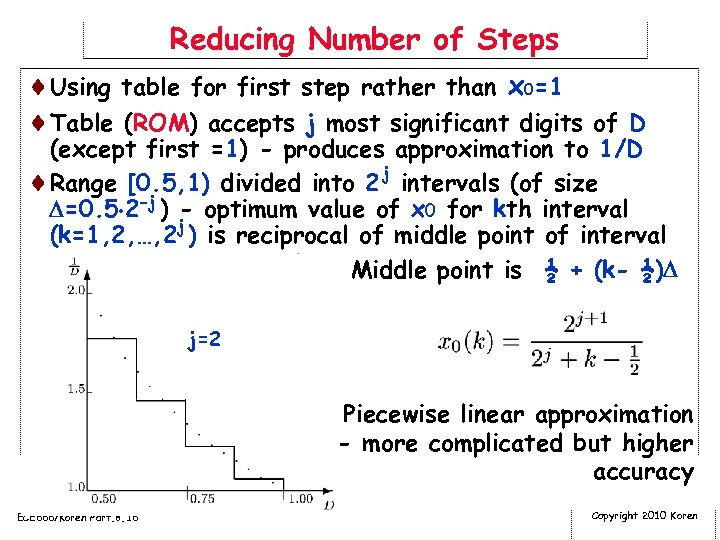Reducing Number of Steps ¨Using table for first step rather than x 0=1 ¨Table (ROM) accepts j most significant digits of D (except first =1) - produces approximation to 1/D ¨Range [0. 5, 1) divided into 2 j intervals (of size =0. 5 2 -j ) - optimum value of x 0 for kth interval (k=1, 2, …, 2 j ) is reciprocal of middle point of interval ¨ Middle point is ½ + (k- ½) j=2 Piecewise linear approximation - more complicated but higher accuracy ECE 666/Koren Part. 8. 16 Copyright 2010 Koren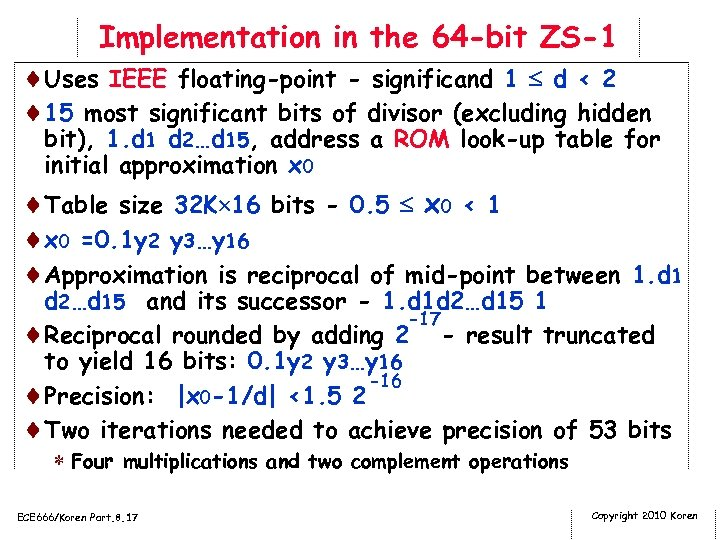Implementation in the 64 -bit ZS-1 ¨Uses IEEE floating-point - significand 1 d < 2 ¨ 15 most significant bits of divisor (excluding hidden bit), 1. d 1 d 2…d 15, address a ROM look-up table for initial approximation x 0 ¨Table size 32 K 16 bits - 0. 5 x 0 < 1 ¨x 0 =0. 1 y 2 y 3…y 16 ¨Approximation is reciprocal of mid-point between 1. d 1 d 2…d 15 and its successor - 1. d 1 d 2…d 15 1 -17 ¨Reciprocal rounded by adding 2 - result truncated to yield 16 bits: 0. 1 y 2 y 3…y 16 -16 ¨Precision: |x 0 -1/d| <1. 5 2 ¨Two iterations needed to achieve precision of 53 bits * Four multiplications and two complement operations ECE 666/Koren Part. 8. 17 Copyright 2010 Koren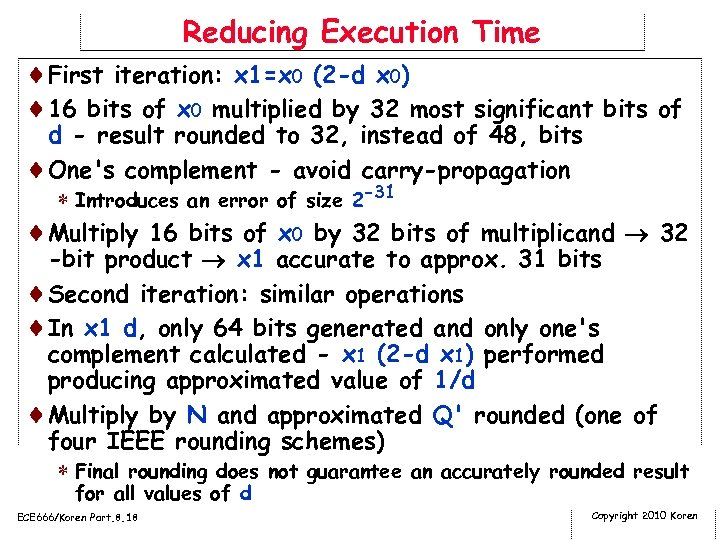Reducing Execution Time ¨First iteration: x 1=x 0 (2 -d x 0) ¨ 16 bits of x 0 multiplied by 32 most significant bits of d - result rounded to 32, instead of 48, bits ¨One's complement - avoid carry-propagation * Introduces an error of size 2 -31 ¨Multiply 16 bits of x 0 by 32 bits of multiplicand 32 -bit product x 1 accurate to approx. 31 bits ¨Second iteration: similar operations ¨In x 1 d, only 64 bits generated and only one's complement calculated - x 1 (2 -d x 1) performed producing approximated value of 1/d ¨Multiply by N and approximated Q' rounded (one of four IEEE rounding schemes) * Final rounding does not guarantee an accurately rounded result for all values of d ECE 666/Koren Part. 8. 18 Copyright 2010 Koren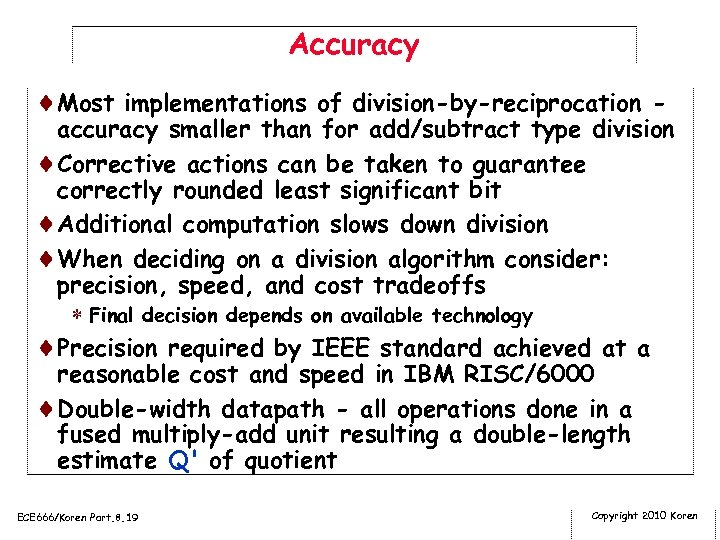Accuracy ¨Most implementations of division-by-reciprocation - accuracy smaller than for add/subtract type division ¨Corrective actions can be taken to guarantee correctly rounded least significant bit ¨Additional computation slows down division ¨When deciding on a division algorithm consider: precision, speed, and cost tradeoffs * Final decision depends on available technology ¨Precision required by IEEE standard achieved at a reasonable cost and speed in IBM RISC/6000 ¨Double-width datapath - all operations done in a fused multiply-add unit resulting a double-length estimate Q' of quotient ECE 666/Koren Part. 8. 19 Copyright 2010 Koren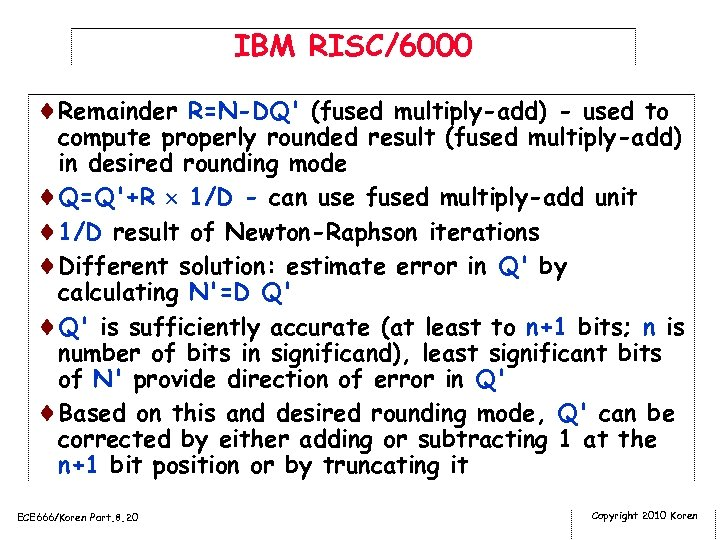IBM RISC/6000 ¨Remainder R=N-DQ' (fused multiply-add) - used to compute properly rounded result (fused multiply-add) in desired rounding mode ¨Q=Q'+R 1/D - can use fused multiply-add unit ¨ 1/D result of Newton-Raphson iterations ¨Different solution: estimate error in Q' by calculating N'=D Q' ¨Q' is sufficiently accurate (at least to n+1 bits; n is number of bits in significand), least significant bits of N' provide direction of error in Q' ¨Based on this and desired rounding mode, Q' can be corrected by either adding or subtracting 1 at the n+1 bit position or by truncating it ECE 666/Koren Part. 8. 20 Copyright 2010 Koren